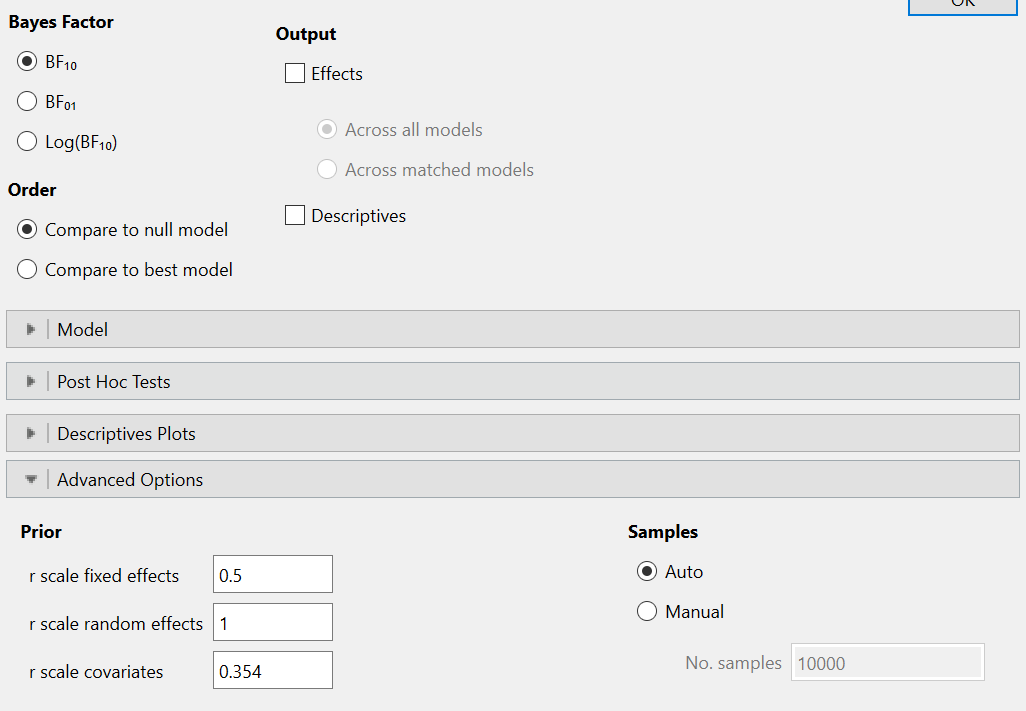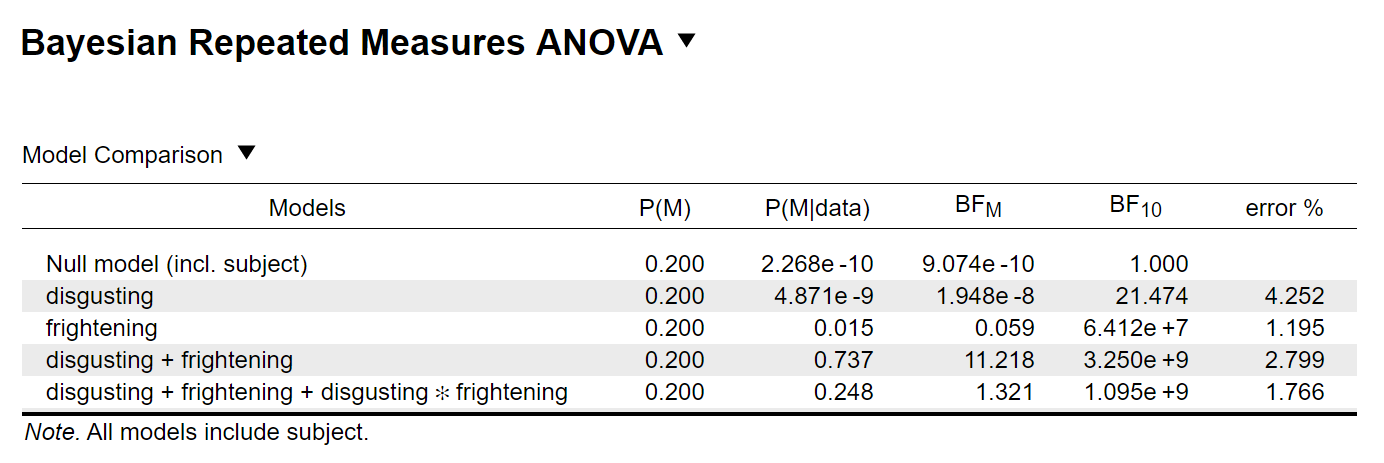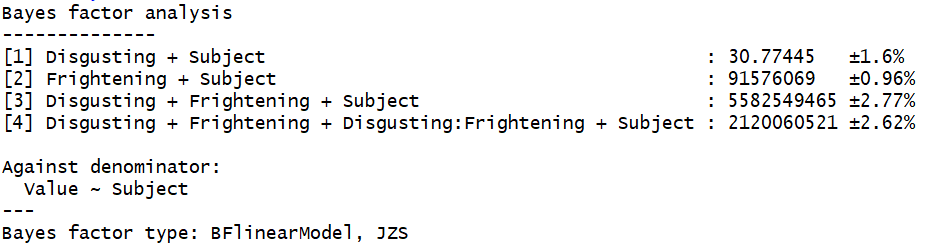#### Howdy, Stranger!

It looks like you're new here. If you want to get involved, click one of these buttons!

Supported by

# Discrepancy Results JASP & BayesFactor (R)

Hello,

I am doing a Bayesian RM-ANOVA with both JASP and the BayesFactor package in R. I am using the example data set - Bugs (Ryan, Wilde & Crist, 2013) - for RM-ANOVA, provided by JASP. I am only using 2 (Disgusting) X 2 (Frightening) as the repeated measures variables. For some reason I get rather different results in JASP and in R.

In JASP I kept the default settings:I get the following output:In R, I first put the data into the long format and excluded missing values (because BayesFactor throws an error otherwise). You can see the script here:

library(tidyverse)
library(BayesFactor)

data <- read_csv(file = "Bugs (Ryan, Wilde & Crist, 2013).csv")

data_cl <- data %>%
gather(key = "key", value = "Value", 5:8) %>%
separate(col = "key", into = c("Disgusting", "Frightening"), sep = ", ") %>%
filter(!(is.na(Value))) %>%
mutate_at(c("Subject", "Disgusting", "Frightening"), as.factor) %>%
as.data.frame()

mod_bay <- anovaBF(formula = Value ~ Disgusting * Frightening + Subject,
data = data_cl,
whichRandom = "Subject")

For the R analysis I get the following results:I am aware that the results are always a bit different due to Monte Carlo sampling. However, I do not think that this explains such large discrepancies in the Bayes factors between JASP and R.
Does someone have an idea how this comes about?

Thank you very much for you help!

• JASP ought to use the BayesFactor code. My first suggestion would be to run the analysis multiple times to get an indication of the variability in the outcomes. How systematic is it?
E.J.